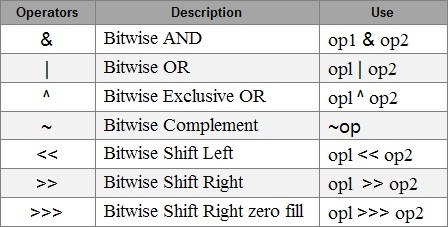# Everything You Need to Know about Bitwise Operators in JavaThe Bitwise operators are used to perform manipulation of individual bits of a number which is an essential aspect of any programming language as ultimately everything comes down to 0 and 1. The following pointers will be covered in this Bitwise Operators in Java article:

Often times, programmers find the need to manipulate numbers. Individual bits of numbers can be modified or manipulated by using the bitwise operators provided by java. These operators can be used with char, short, int or any of the integral types. They cannot be applied to double and float.## Types of Bitwise Operators in Java

• & (Binary AND Operator)

The Binary & operators are very much similar to the logical && operators, the only difference being they work with two bits instead of two expressions. The Binary AND operator returns the value 1 is both the operands are equal to one, else they return 0.

• | ( Binary OR Operator)

The Binary OR operator is similar to the logical || operator. It works on two bits instead of two expressions and returns 1 if either one of its operands evaluates as 1. The result is 1 even if both the operands evaluate to 1.

• ^ ( Binary XOR Operator)

XOR stand for “exclusive OR”. This operator returns 1, if exactly one of its operands evaluate to 1. The result is 0, if both the operands evaluate to 1 or 0.

• ~( Binary Complement Operator)

The one’s complement of the input value is returned by this operator. In simpler terms, it inverses the bits i.e. it converts the 0’s to 1’s and vice versa.

## Examples of Bitwise Operators in Java

```public class bitwiseExample {
public static void main(String[] args) {
//Variables Definition and Initialization
int n1 = 18, n2 = 28, n3 =0;
//Bitwise AND
System.out.println("num1 & num2 = " + (n1 & n2));
//Bitwise OR
System.out.println("num1 | num2 = " + (n1 | n2) );
//Bitwise XOR
System.out.println("num1 ^ num2 = " + (n1 ^ n2) );
//Binary Complement Operator
System.out.println("~num1 = " + ~n1 );
}
}

```

Output:

num1 & num2 = 16

num1 | num2 = 30

num1 ^ num2 = 14

~num1 = -19

## Shift Operators

These operators shift the numbers either to the left or right, multiplying and dividing the numbers respectively.

• >>( Signed Right Shift Operator):

This operator shifts the number to the right. It fills 0 in the empty spaces that are left as a result. The leftmost bit is dependent upon the sign of the initial number. Similar to dividing a number with some power of two.

• >>>( Unsigned Right Shift Operator):

This operator shifts the number to the right. It fills 0 in the empty spaces that are left as a result. The leftmost bit is set to 0.

• >>( Left Shift Operator):

This operator shifts the number to the left. It fills 0 in the empty spaces that are left as a result. Similar to multiplying a number with some power of two.

• >>( Unsigned Left Shift Operator):

Java does not provide any such operator, unlike the unsigned right shift.

Example of Shift Operator

```public class bitwiseExample {
public static void main(String[] args)
{
int n1 = 8;
int n2 = -10;
// left shift operator
System.out.println("n1<<2 = " + (n1 << 2)); // right shift operator System.out.println("n2>>2 = " + (n2 >> 2));
// unsigned right shift operator
System.out.println("n2>>>2 = " + (n2 >>> 2));
}
}

```

Output:

n1<<2 = 32

n2>>2 = -3

n2>>>2 = 1073741821

With this, we come to an end of this Bitwise Operators in Java article. The operators discussed in the article allow the user to manipulate the numbers or individual bits of data effectively. Check out the Java training by Edureka, a trusted online learning company with a network of more than 250,000 satisfied learners spread across the globe. Edureka’s Java J2EE and SOA training and certification course is designed for students and professionals who want to be a Java Developer. The course is designed to give you a head start into Java programming and train you for both core and advanced Java concepts along with various Java frameworks like Hibernate & Spring.

Got a question for us? Please mention it in the comments section of this “Bitwise Operators in Java” blog  and we will get back to you as soon as possible.

Upcoming Batches For Java Certification Training Course
Course NameDate
Java Certification Training Course

Class Starts on 25th February,2023

25th February

SAT&SUN (Weekend Batch)
View Details
Java Certification Training Course

Class Starts on 25th March,2023

25th March

SAT&SUN (Weekend Batch)
View DetailsREGISTER FOR FREE WEBINARThank you for registering Join Edureka Meetup community for 100+ Free Webinars each month

### Subscribe to our Newsletter, and get personalized recommendations.#### Everything You Need to Know about Bitwise Operators in Java

edureka.co